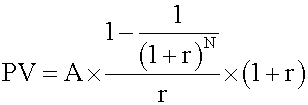Tags
Question
Present value of an annuity due

(without breaking it in 2)Tags
Question
Present value of an annuity due

(without breaking it in 2)

?

Tags
Question
Present value of an annuity due

(without breaking it in 2)If you want to change selection, open document below and click on "Move attachment"

Subject 4. The Future Value and Present Value of a Series of Equal Cash Flows (Ordinary Annuities, Annuity Dues, and Perpetuities)
u can use this formula: Note that, all other factors being equal, the future value of an annuity due is equal to the future value of an ordinary annuity multiplied by (1 + r). <span>Present value of an annuity due This consists of two parts: an annuity payment now and the present value of a regular annuity of (N - 1) period. Use the above formula to calculate the second part and add the two parts together. This process can also be simplified to a formula: Note that, all other factors being equal, the present value of an annuity due is equal to the present value of an ordinary annuity multiplied

#### Summary

status measured difficulty not learned 37% [default] 0

No repetitions